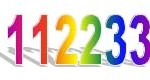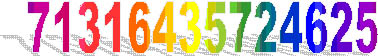Suppose you have two 1s, two 2s and two 3s.
Arrange these six digits in a list so that:

between the two 1s there is one digit giving 1?1,
between the two 2s there are two digits giving 2??2,
between the two 3s there are three digits giving 3???3.

Can you do the same if you only have 1s and 2s? Explain your answer.

Can you do the same if you include two fours, and between the two 4s there are four digits?

The illustration shows a 7-sandwich using the pairs of all the whole numbers from 1 to 7.

Can you make another 7-sandwich?For some values of n it is possible to make n-sandwiches using pairs of every positive integer from 1 to n, and arranging the list according to the rules given above, and for other values of n it is not possible. The big challenge is to find when it is and when it is not possible.

See the Notes for Teachers  and you may like to use this Puzzle Sheet.